#### 3D triangles

Suppose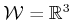. One of the most convenient geometric models to express is a set of triangles, each of which is specified by three points,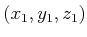,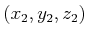,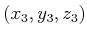. This model has been popular in computer graphics because graphics acceleration hardware primarily uses triangle primitives. It is assumed that the interior of the triangle is part of the model. Thus, two triangles are considered as colliding'' if one pokes into the interior of another. This model offers great flexibility because there are no constraints on the way in which triangles must be expressed; however, this is also one of the drawbacks. There is no coherency that can be exploited to easily declare whether a point is inside'' or outside'' of a 3D obstacle. If there is at least some coherency, then it is sometimes preferable to reduce redundancy in the specification of triangle coordinates (many triangles will share the same corners). Representations that remove this redundancy are called a triangle strip, which is a sequence of triangles such that each adjacent pair shares a common edge, and a triangle fan, which is a triangle strip in which all triangles share a common vertex. See Figure 3.6.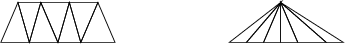Steven M LaValle 2020-08-14